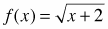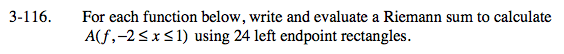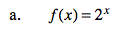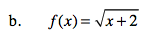### Home > CALC > Chapter 3 > Lesson 3.3.3 > Problem3-116

3-116.
1. For each function below, write and evaluate a Riemann sum to calculate A(f, −2 ≤ x ≤ 1) using 24 left endpoint rectangles. Homework Help ✎

1. f(x) = 2x

2.General form of a left-endpoint Riemann sum:where a and b are the starting and ending values and n is the number of rectangles.Refer to the hints in part (a).